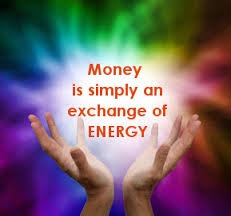# Money as an Idea-4-Energy

## Money as an Idea-4-Energy

### MONEY IS ENERGY

When money flows, it becomes energy.  When electricity flows, it provides energy.
The only reason that money is powerful is because it is dynamic.  Money is active.
The more dynamic, the more powerful.A booming economy is dynamic.
An economy in recession has less activity.
Hope activates the economy.
When interest rates drop, economic activity increases.

I would like to introduce a couple of ideas about money.
In order to do this, let’s consider some basics in physics.
When an object moves, it has mass (weight), direction of movement, velocity (speed) in that direction, momentum, energy and power.
There are formulae that relate these aspects.

I propose that the same is true for money.
In order to recognize this, it helps to let go of your fears regarding money.  Fear does not allow you to see money truly.
I want the power associated with money, not just the weight.
Better understanding generally helps alleviate fears regarding money.
To better understand, let’s looks at some physics relationships.

As mass doubles (and speed is constant):
Momentum doubles
Energy doubles
Power doubles

As speed doubles (and mass is constant):
Momentum doubles

If mass and speed both double:
Momentum is multiplied by four
Energy is multiplied by eight
Power is multiplied by eight

Like I said, I want the power associated with money, not just the weight.
Therefore, I want my money to be as fast as possible.  What does that mean?

Here are some questions I like to ask.
How quickly does my investment prosper?
How quickly does my money move from one investment to another?
What is the interest rate or the rate of return on investment?
What is the cash flow?
These questions illustrate the speed of money.

To have energy and power, money must flow.  The faster it flows, the more energy and power.  If you multiply the speed of money by 10, you multiply the energy and power of that money by 100.  But what if the flow stops or slows?  If there is a blockage of the flow of money (same as slowing the flow of water), it loses its energy.  Two forces that can block the flow of money are fear and greed.  On the spiritual side of things, the opposite of abundance is greed.  Here is an analogy for this.  When my hands are full, I cannot receive anything.

### Who will you be when you unlock your potential?